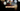# Leetcode | Solution of Day of Week in JavaScript

April 4th, 2020
|

In this post, we will solve the day of the week from leetcode and compute it's time and space complexities. Let's begin.

# Problem Statement

The question can be found at leetcode day of week problem.

The problem states that we are given a date and we need to identify which day of the week falls on that particular date.

# Solution

The approach for this one is pretty simple. We are given a date, so we can use the `Date` function from JavaScript and get the actual day. The only problem is the day is represented as numbers from `0 to 6` for `Sunday through Saturday`. So, we'll keep an array to keep our days and return the day at the index returned from the date function.

We have discussed the approach, I urge you to go ahead on leetcode and give it another try.If you are here, it means something went wrong in implementation or you are just too lazy. In any case, let's see a simple implementation of the above logic.
``````var dayOfTheWeek = function (day, month, year) {
const days = ["Sunday", "Monday", "Tuesday", "Wednesday", "Thursday", "Friday", "Saturday"]

return days[(new Date(year, month - 1, day)).getDay()]
};``````

The solution is very straight forward. We use the date in the arguments to get the day using JavaScript `Date` function. Notice, the month is subtracted by `1`, because, javascript dates start from `0` as opposed to `1`. Next, we return the day at the index returned from the date function.

Here are the stats on submission

``````Status: Accepted
Runtime: 120ms
Memory: 33.5MB``````

## Time and space complexity

### Time complexity

We are only doing constant operations, so time complexity would be O(1).

### Space complexity

We are using extra space only to store the days in an array, but the length of the array is constant. So space complexity is constant, O(1).

# Summary

So, we solved the day of week problem using JavaScript Date function and calculated the time and space complexities.

I hope you enjoyed solving this question. This is it for this one, complete source code for this post can be found on my Github Repo. Will see you in the next one.

There you go guys, you made it to end of the post. Subscribe to my youtube channel for regular updates. Follow me on twitter, drop me a mail or leave a comment here if you still have any doubts and I will try my best to help you out. Thanks

Stay tuned and see you around :)